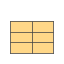Edge With Initial Value - MapleSim Help

Edge With Init

Indicates rising edge of Boolean signalDescription The Boolean output is $\mathrm{true}$ when the Boolean input has a rising edge from $\mathrm{false}$ to $\mathrm{true}$, otherwise the output is $\mathrm{false}$. The output is $\mathrm{true}$ only for an instant, so a plot of it will always appear $\mathrm{false}$.Equations $y=\mathrm{edge}\left(u\right)$Connections

 Name Description Modelica ID $u$ Boolean input signal u $y$ Boolean output signal yParameters

 Name Default Units Description Modelica ID ${\mathrm{pre}}_{{u}_{0}}$ $\mathrm{false}$ Start value of pre(u) at initial time pre_u_startModelica Standard Library The component described in this topic is from the Modelica Standard Library. To view the original documentation, which includes author and copyright information, click here.